International
Tables for
Crystallography
Volume B
Reciprocal space
Edited by U. Shmueli

International Tables for Crystallography (2010). Vol. B, ch. 4.6, pp. 619-621   | 1 | 2 |

## Section 4.6.3.3.3.5. Relationships between structure factors at symmetry-related points of the Fourier image

W. Steurera* and T. Haibacha

aLaboratory of Crystallography, Department of Materials, ETH Hönggerberg, HCI G 511, Wolfgang-Pauli-Strasse 10, CH-8093 Zurich, Switzerland
Correspondence e-mail:  walter.steurer@mat.ethz.ch

#### 4.6.3.3.3.5. Relationships between structure factors at symmetry-related points of the Fourier image

| top | pdf |

The weighted 3D reciprocal space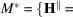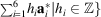exhibits the icosahedral point symmetry. It is invariant under the action of the scaling matrix: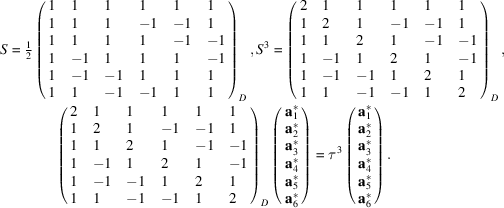The scaling transformationleaves a primitive 6D reciprocal lattice invariant as can easily be seen from its application on the indices:The matrixleavesinvariant,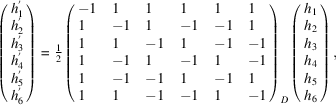for anywithall even or all odd, corresponding to a 6D face-centred hypercubic lattice. In a second case the sumis even, corresponding to a 6D body-centred hypercubic lattice. Block-diagonalization of the matrix S decomposes it into two irreducible representations. Withwe obtain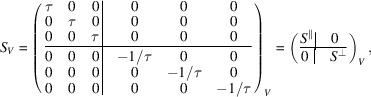the scaling properties in the two 3D subspaces: scaling by a factor τ in parallel space corresponds to a scaling by a factorin perpendicular space. For the intensities of the scaled reflections analogous relationships are valid, as discussed for decagonal phases (Figs. 4.6.3.36and 4.6.3.37, Section 4.6.3.3.2.5).Figure 4.6.3.36 | top | pdf |Parallel-space distribution of (a) positive and (b) negative structure factors of the 3D Penrose tiling of the 6D P lattice type decorated with point atoms (edge lengths of the Penrose unit rhombohedra ar = 5.0 Å). The magnitudes of the structure factors are indicated by the diameters of the filled circles. All reflections are shown withinand.Figure 4.6.3.37 | top | pdf |Perpendicular-space distribution of (a) positive and (b) negative structure factors of the 3D Penrose tiling of the 6D P lattice type decorated with point atoms (edge lengths of the Penrose unit rhombohedra ar = 5.0 Å). The magnitudes of the structure factors are indicated by the diameters of the filled circles. All reflections are shown withinand.